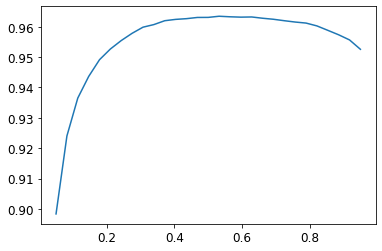# Should We Retrain the Model after getting the Best Threshold for MultiCategory?

Question Regarding `06_mutlicat.ipynb`

In the lesson, there’s a part we are trying to set the best thresh to use with `accuracy_multi`

The initial learner was created by:

``````learn = cnn_learner(dls, resnet50, metrics=partial(accuracy_multi, thresh=0.2))
``````

and it gave us a final validation multi accuracy of 0.95

First we try:

``````learn.metrics = partial(accuracy_multi, thresh=0.1)
learn.validate()
``````

which gives us 0.93

then:

``````learn.metrics = partial(accuracy_multi, thresh=0.99)
learn.validate()
``````

gives us 0.94

Instead of trial and error, Jeremy suggests we use:

``````preds,targs = learn.get_preds()

xs = torch.linspace(0.05,0.95,29)
accs = [accuracy_multi(preds, targs, thresh=i, sigmoid=False) for i in xs]
plt.plot(xs,accs);
``````

which gives us thisSo the best thresh would be around 0.5 - 0.6

Here’s my question, after we get this thresh should we create a new learner and re-run the training of the model using this syntax:

``````learn = cnn_learner(dls, resnet50, metrics=partial(accuracy_multi, thresh=0.5))
learn.fine_tune(3, base_lr=3e-3, freeze_epochs=4)
``````

Or does this method that we were using earlier change the threshold of the learner and that new threshold will be used when I export the model?

``````learn.metrics = partial(accuracy_multi, thresh=0.5)
``````

In short, does changing the metrics of a Learner warrant a retrain?

Yes but something very important here. You need to set the loss functions threshold too, otherwise it will be training with .5

So loss_func = BCELossLogitsFlat(thresh=thresh)

And then pass that loss function into cnn_learner along with the rest of it

Thanks! @muellerzr. I seriously didn’t know the loss functions use thresholds too. I will update accordingly

Is this provided by fastai?
Because the one from PyTorch `nn.BCEWithLogitsLoss()` doesn’t seem to have a parameter called thresh?

Im getting this output:

TypeError: init() got an unexpected keyword argument ‘thresh’

and running

``````BCELossLogitsFlat(thresh=0.5)
``````

results in:

NameError: name ‘BCELossLogitsFlat’ is not defined

Couldn’t quite remember what fastai’s loss function wastry it with BCEWithLogitsLossFlat

1 Like